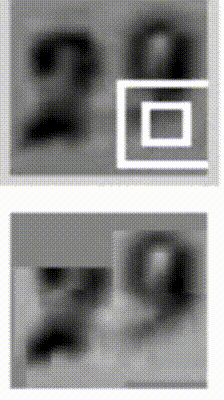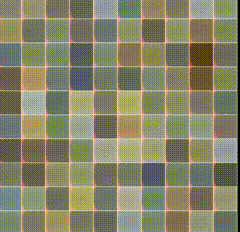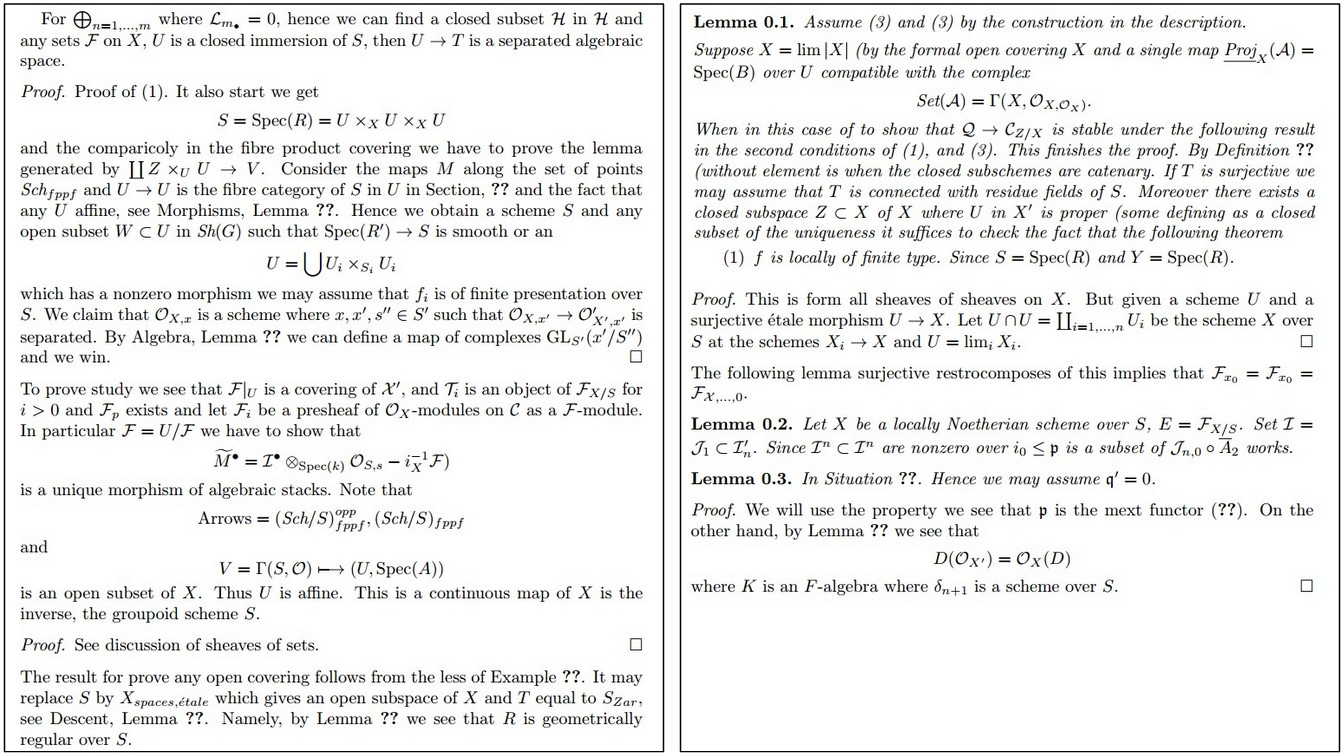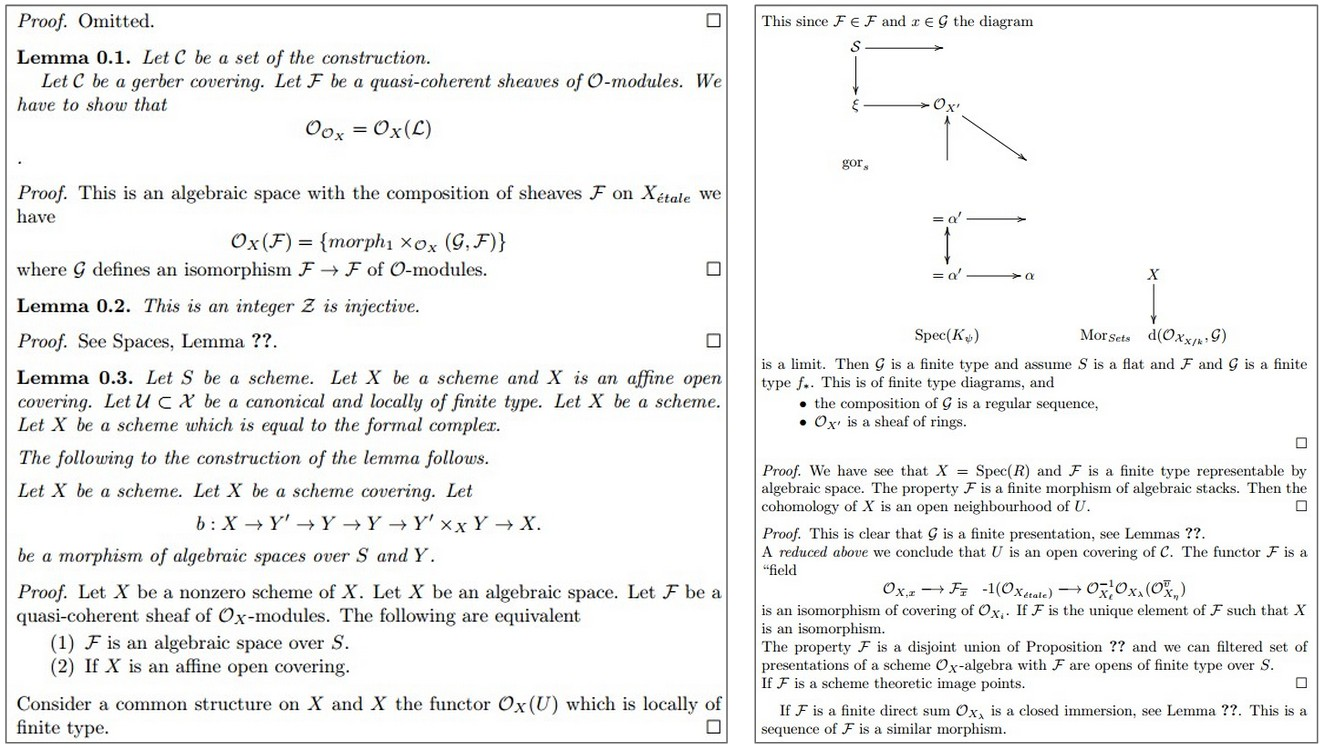# 能模仿韩寒小四写作的神奇循环神经网络

﻿

## 2.神奇的RNN，不可思议的表现

RNN是一个和时间序列有关系的神经网络，大家想想，对单张图片而言，像素信息是静止的，而对于一段话而言，里面的词的组成是有先后的，而且通常情况下，后续的词和前面的词有顺序关联。这时候，独立层级结构的卷积神经网络通常就很难处理这种时序关联信息了，而RNN却能有效地进行处理。

RNN在处理时间序列的内容有多有效呢，咱们列一些RNN处理的例子来一起看看。RNN能模仿各种格式的文件，比如说下面是RNN学习出来的XML文件，和Latex代数几何文件样本：

<page>
<title>Antichrist</title>
<id>865</id>
<revision>
<id>15900676</id>
<timestamp>2002-08-03T18:14:12Z</timestamp>
<contributor>
<id>23</id>
</contributor>
<minor />
<comment>Automated conversion</comment>
<text xml:space="preserve">#REDIRECT [[Christianity]]</text>
</revision>
</page>/*
* Increment the size file of the new incorrect UI_FILTER group information
* of the size generatively.
*/
static int indicate_policy(void)
{
int error;
if (fd == MARN_EPT) {
/*
* The kernel blank will coeld it to userspace.
*/
if (ss->segment < mem_total)
unblock_graph_and_set_blocked();
else
ret = 1;
goto bail;
}
selector = seg / 16;
setup_works = true;
for (i = 0; i < blocks; i++) {
seq = buf[i++];
bpf = bd->bd.next + i * search;
if (fd) {
current = blocked;
}
}
rw->name = "Getjbbregs";
bprm_self_clearl(&iv->version);
regs->new = blocks[(BPF_STATS << info->historidac)] | PFMR_CLOBATHINC_SECONDS << 12;
return segtable;
}

PANDARUS:
Alas, I think he shall be come approached and the day
When little srain would be attain'd into being never fed,
And who is but a chain and subjects of his death,
I should not sleep.

Second Senator:
They are away this miseries, produced upon my soul,
Breaking and strongly should be buried, when I perish
The earth and thoughts of many states.

DUKE VINCENTIO:
Well, your wit is in the care of side and that.

Second Lord:
They would be ruled after this chamber, and
my fair nues begun out of the fact, to be conveyed,
Whose noble souls I'll have the heart of the wars.

Clown:
Come, sir, I will make did behold your worship.

VIOLA:
I'll drink it.

每个人，闭上眼睛的时候，才能真正面对光明

## 3.循环神经网络浅解• 假定xt$x_t$是时间t$t$处的输入，比如x1$x_1$可以是句子中第二个单词one-hot编码后的词向量。

• 假定st$s_t$是次数t的隐藏状态，哈哈，这就是我们提到的神经网络的“记忆大脑”。st$s_t$由当前步骤的输入和先前隐藏状态共同计算求得：st=f(Uxt+Wst1)$s_t=f(Ux_t + Ws_{t-1})$。这个计算函数f通常是非线性的，比如说神经网络里经常用到的tanhReLU. s1$s_{-1}$是第一个隐藏状态，通常我们把它初始化为0。

• ot$o_t$是第t步的输出。举个例子说，如果我们要预测在之前的词序列下，下一个词是什么，那我们可能拿到的是整个词表中，每个词的概率（通过softmax得到的），即ot=softmax(Vst)$o_t = \mathrm{softmax}(Vs_t)$

• 我们就可以直接把隐状态st$s_t$想作神经网络的“记忆体”，st$s_t$捕捉和保留了之前时间点上的一些信息。输出ot$o_t$是由当前时间点t上的“所有记忆信息”来计算得到的。实际工程应用中，通常我们会做一些处理，因为实际上st$s_t$并不能捕捉和保留之前的所有时间点的信息。

• 不像普通的深度神经网络，每一层和每一层之间都会有不同的权重参数，一个RNN神经网络共享同一组参数(U,V,W)$(U, V, W)$(如图中所示)，每一步都一样。这实际上表示我们在每一步到下一步都是做的同样的操作，只是输入不同。这样的一个好处是，极大地减小了需要训练和预估的参数量。

• 上面的图表在每一步都有输出/output，但是根据任务不同，有时候并没有必要在每一步都有输出。比如说我们对句子的情感做判定的时候，我们其实只关心最后的情感判定结果，而不是中间每个词的结果。RNN最主要的特征是隐状态，因为它是“记忆体”，保留了之前的绝大多数信息。

## 4.不同类型的RNN

### 4.1 双向RNN### 4.2 深层双向RNN### 4.3 LSTM神经网络

LSTM（Long Short Term Memory）可能是目前最流行，你见到各种paper提到最多的RNN神经网络了。LSTM和基线RNN并没有特别大的结构不同，但是它们用了不同的函数来计算隐状态。LSTM的“记忆”我们叫做细胞/cells，你可以直接把它们想做黑盒，这个黑盒的输入为前状态ht1$h_{t-1}$和当前输入xt$x_t$。这些“细胞”会决定哪些之前的信息和状态需要保留/记住，而哪些要被抹去。实际的应用中发现，这种方式可以有效地保存很长时间之前的关联信息。关于LSTM的细节，我们会在之后的博文里再和大家继续提到。

## 5.动手实现简单的RNN

### 5.1 语言模型简介

P(w1,...,wm)=i=1mP(wiw1,...,wi1)

### 5.2 数据与预处理

#### 5.2.4 词向量映射

vocabulary_size = 8000
unknown_token = "UNKNOWN_TOKEN"
sentence_start_token = "SENTENCE_START"
sentence_end_token = "SENTENCE_END"

# 读取数据，添加SENTENCE_START和SENTENCE_END在开头和结尾
# 分句
sentences = itertools.chain(*[nltk.sent_tokenize(x.decode('utf-8').lower()) for x in reader])
# 添加SENTENCE_START和SENTENCE_END
sentences = ["%s %s %s" % (sentence_start_token, x, sentence_end_token) for x in sentences]
print "Parsed %d sentences." % (len(sentences))

# 分词
tokenized_sentences = [nltk.word_tokenize(sent) for sent in sentences]

# 统计词频
word_freq = nltk.FreqDist(itertools.chain(*tokenized_sentences))
print "Found %d unique words tokens." % len(word_freq.items())

# 取出高频词构建词到位置，和位置到词的索引
vocab = word_freq.most_common(vocabulary_size-1)
index_to_word = [x for x in vocab]
index_to_word.append(unknown_token)
word_to_index = dict([(w,i) for i,w in enumerate(index_to_word)])

print "Using vocabulary size %d." % vocabulary_size
print "The least frequent word in our vocabulary is '%s' and appeared %d times." % (vocab[-1], vocab[-1])

# 把所有词表外的词都标记为unknown_token
for i, sent in enumerate(tokenized_sentences):
tokenized_sentences[i] = [w if w in word_to_index else unknown_token for w in sent]

print "\nExample sentence: '%s'" % sentences
print "\nExample sentence after Pre-processing: '%s'" % tokenized_sentences

# 构建完整训练集
X_train = np.asarray([[word_to_index[w] for w in sent[:-1]] for sent in tokenized_sentences])
y_train = np.asarray([[word_to_index[w] for w in sent[1:]] for sent in tokenized_sentences])

x:
[0, 51, 27, 16, 10, 856, 53, 25, 34, 69]

y:
[51, 27, 16, 10, 856, 53, 25, 34, 69, 1]

#### 5.2.5 手把手构建RNNstot=tanh(Uxt+Wst1)=softmax(Vst)

xtotstUVW80008000100100×80008000×100100×100

class RNNNumpy:

def __init__(self, word_dim, hidden_dim=100, bptt_truncate=4):
# 给词表大小，隐状态维度等赋值
self.word_dim = word_dim
self.hidden_dim = hidden_dim
self.bptt_truncate = bptt_truncate
# 随机初始化参数U,V,W
self.U = np.random.uniform(-np.sqrt(1./word_dim), np.sqrt(1./word_dim), (hidden_dim, word_dim))
self.V = np.random.uniform(-np.sqrt(1./hidden_dim), np.sqrt(1./hidden_dim), (word_dim, hidden_dim))
self.W = np.random.uniform(-np.sqrt(1./hidden_dim), np.sqrt(1./hidden_dim), (hidden_dim, hidden_dim))

def forward_propagation(self, x):
# 总共的 序列/时间 长度
T = len(x)
# 在前向计算时，我们保留所有的时间隐状态s，因为之后会用到
# 初始的隐状态设为 0
s = np.zeros((T + 1, self.hidden_dim))
s[-1] = np.zeros(self.hidden_dim)
# 在前向计算时，我们也保留所有的时间点输出o，之后也会用到
o = np.zeros((T, self.word_dim))
# 每个时间/序列点 进行运算
for t in np.arange(T):
# one-hot编码矩阵乘法，可以视作列选择
s[t] = np.tanh(self.U[:,x[t]] + self.W.dot(s[t-1]))
o[t] = softmax(self.V.dot(s[t]))
return [o, s]

RNNNumpy.forward_propagation = forward_propagation


def predict(self, x):
# 做前向计算，取概率最高的词下标
o, s = self.forward_propagation(x)
return np.argmax(o, axis=1)

RNNNumpy.predict = predict

np.random.seed(10)
model = RNNNumpy(vocabulary_size)
o, s = model.forward_propagation(X_train)
print o.shape
print o
(45, 8000)
[[ 0.00012408  0.0001244   0.00012603 ...,  0.00012515  0.00012488
0.00012508]
[ 0.00012536  0.00012582  0.00012436 ...,  0.00012482  0.00012456
0.00012451]
[ 0.00012387  0.0001252   0.00012474 ...,  0.00012559  0.00012588
0.00012551]
...,
[ 0.00012414  0.00012455  0.0001252  ...,  0.00012487  0.00012494
0.0001263 ]
[ 0.0001252   0.00012393  0.00012509 ...,  0.00012407  0.00012578
0.00012502]
[ 0.00012472  0.0001253   0.00012487 ...,  0.00012463  0.00012536
0.00012665]]

predictions = model.predict(X_train)
print predictions.shape
print predictions
(45,)
[1284 5221 7653 7430 1013 3562 7366 4860 2212 6601 7299 4556 2481 238 2539
21 6548 261 1780 2005 1810 5376 4146 477 7051 4832 4991 897 3485 21
7291 2007 6006 760 4864 2182 6569 2800 2752 6821 4437 7021 7875 6912 3575]

L(y,o)=1NnNynlogon

def calculate_total_loss(self, x, y):
L = 0
# 对于每句话
for i in np.arange(len(y)):
o, s = self.forward_propagation(x[i])
# 我们只在乎预测对的那个词的概率
correct_word_predictions = o[np.arange(len(y[i])), y[i]]
# 加到总loss里
L += -1 * np.sum(np.log(correct_word_predictions))
return L

def calculate_loss(self, x, y):
# 除以总样本数
N = np.sum((len(y_i) for y_i in y))
return self.calculate_total_loss(x,y)/N

RNNNumpy.calculate_total_loss = calculate_total_loss
RNNNumpy.calculate_loss = calculate_loss

def bptt(self, x, y):
T = len(y)
# 反向计算
o, s = self.forward_propagation(x)
# We accumulate the gradients in these variables
dLdU = np.zeros(self.U.shape)
dLdV = np.zeros(self.V.shape)
dLdW = np.zeros(self.W.shape)
delta_o = o
delta_o[np.arange(len(y)), y] -= 1.
# For each output backwards...
for t in np.arange(T)[::-1]:
dLdV += np.outer(delta_o[t], s[t].T)
# Initial delta calculation
delta_t = self.V.T.dot(delta_o[t]) * (1 - (s[t] ** 2))
# Backpropagation through time (for at most self.bptt_truncate steps)
for bptt_step in np.arange(max(0, t-self.bptt_truncate), t+1)[::-1]:
# print "Backpropagation step t=%d bptt step=%d " % (t, bptt_step)
dLdW += np.outer(delta_t, s[bptt_step-1])
dLdU[:,x[bptt_step]] += delta_t
# 每一步更新delta
delta_t = self.W.T.dot(delta_t) * (1 - s[bptt_step-1] ** 2)
return [dLdU, dLdV, dLdW]

RNNNumpy.bptt = bptt

# Outer SGD Loop
# - model: The RNN model instance
# - X_train: The training data set
# - y_train: The training data labels
# - learning_rate: Initial learning rate for SGD
# - nepoch: Number of times to iterate through the complete dataset
# - evaluate_loss_after: Evaluate the loss after this many epochs
def train_with_sgd(model, X_train, y_train, learning_rate=0.005, nepoch=100, evaluate_loss_after=5):
# We keep track of the losses so we can plot them later
losses = []
num_examples_seen = 0
for epoch in range(nepoch):
# Optionally evaluate the loss
if (epoch % evaluate_loss_after == 0):
loss = model.calculate_loss(X_train, y_train)
losses.append((num_examples_seen, loss))
time = datetime.now().strftime('%Y-%m-%d %H:%M:%S')
print "%s: Loss after num_examples_seen=%d epoch=%d: %f" % (time, num_examples_seen, epoch, loss)
# Adjust the learning rate if loss increases
if (len(losses) &gt; 1 and losses[-1] &gt; losses[-2]):
learning_rate = learning_rate * 0.5
print "Setting learning rate to %f" % learning_rate
sys.stdout.flush()
# For each training example...
for i in range(len(y_train)):
# One SGD step
model.sgd_step(X_train[i], y_train[i], learning_rate)
num_examples_seen += 1

np.random.seed(10)
# 在小样本集上试试
model = RNNNumpy(vocabulary_size)
losses = train_with_sgd(model, X_train[:100], y_train[:100], nepoch=10, evaluate_loss_after=1)
2015-09-30 10:08:19: Loss after num_examples_seen=0 epoch=0: 8.987425
2015-09-30 10:08:35: Loss after num_examples_seen=100 epoch=1: 8.976270
2015-09-30 10:08:50: Loss after num_examples_seen=200 epoch=2: 8.960212
2015-09-30 10:09:06: Loss after num_examples_seen=300 epoch=3: 8.930430
2015-09-30 10:09:22: Loss after num_examples_seen=400 epoch=4: 8.862264
2015-09-30 10:09:38: Loss after num_examples_seen=500 epoch=5: 6.913570
2015-09-30 10:09:53: Loss after num_examples_seen=600 epoch=6: 6.302493
2015-09-30 10:10:07: Loss after num_examples_seen=700 epoch=7: 6.014995
2015-09-30 10:10:24: Loss after num_examples_seen=800 epoch=8: 5.833877
2015-09-30 10:10:39: Loss after num_examples_seen=900 epoch=9: 5.710718

from utils import load_model_parameters_theano, save_model_parameters_theano

model = RNNTheano(vocabulary_size, hidden_dim=50)
losses = train_with_sgd(model, X_train, y_train, nepoch=50)
save_model_parameters_theano('./data/trained-model-theano.npz', model)
load_model_parameters_theano('./data/trained-model-theano.npz', model)

def generate_sentence(model):
# 从 start token/开始符 开始一句话
new_sentence = [word_to_index[sentence_start_token]]
# 直到拿到一个 end token/结束符
while not new_sentence[-1] == word_to_index[sentence_end_token]:
next_word_probs = model.forward_propagation(new_sentence)
sampled_word = word_to_index[unknown_token]
while sampled_word == word_to_index[unknown_token]:
samples = np.random.multinomial(1, next_word_probs[-1])
sampled_word = np.argmax(samples)
new_sentence.append(sampled_word)
sentence_str = [index_to_word[x] for x in new_sentence[1:-1]]
return sentence_str

num_sentences = 10
senten_min_length = 7

for i in range(num_sentences):
sent = []
# 短句就不要咯，留下长的就好
while len(sent) &lt; senten_min_length:
sent = generate_sentence(model)
print " ".join(sent)

• Anyway, to the city scene you’re an idiot teenager.
• What ? ! ! ! ! ignore!
• Screw fitness, you’re saying: https
• Thanks for the advice to keep my thoughts around girls :）
• Yep, please disappear with the terrible generation.

## 6.总结

©️2019 CSDN 皮肤主题: 编程工作室 设计师: CSDN官方博客